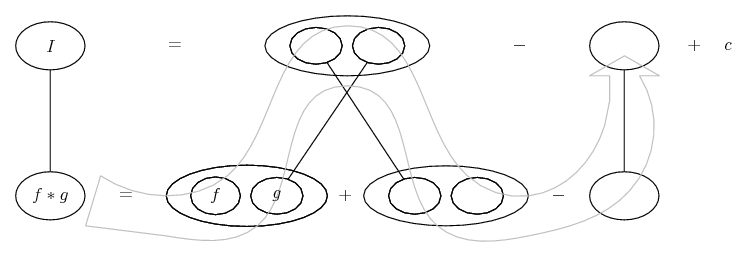# Thread: Integration by Substitution

1. ## Integration by Substitution

Hi, I'm new to integration. Can anyone help me figure out where I went wrong?
Many thanks.

Q. Evaluate the following:

Please see attachment.

2. ## Re: Integration by Substitution

Notice your u version of the integrand has no traces of the x in the numerator of the original. To bring that part with you, you can re-express it (x, that is) in terms of u, or else use integration by parts.

3. ## Re: Integration by SubstitutionOriginally Posted by GrigOrig99Hi, I'm new to integration. Can anyone help me figure out where I went wrong?
Many thanks.

Q. Evaluate the following:

Please see attachment.
Your numerator disappeared when changing the variable. Express x in terms of u.

edit: drat, beaten to it4. ## Re: Integration by Substitution

You haven't taken into account the $\displaystyle x$ on the numerator.

We have:

$\displaystyle \int^4_0{\frac{x}{\sqrt{2x+1}}}dx$

I'm going to let $\displaystyle u^2=2x+1$ so that $\displaystyle 2u~du=2~dx$ and $\displaystyle dx=u~du$

When $\displaystyle x=4$, $\displaystyle u=3$ and when $\displaystyle x=0$, $\displaystyle u=1$

I have:

$\displaystyle \int^4_0{\frac{x}{\sqrt{2x+1}}}dx$

=$\displaystyle \int^3_1{\frac{u^2-1}{2u}}\cdot{u}~du$

Take it!

5. ## Re: Integration by Substitution

The parts version...... where (key in spoiler) ...

Spoiler:... is the product rule, straight continuous lines differentiating downwards with respect to x.... is lazy integration by parts, doing without u and v.

__________________________________________________ __________

Don't integrate - balloontegrate!

Balloon Calculus; standard integrals, derivatives and methods

Balloon Calculus Drawing with LaTeX and Asymptote!

6. ## Re: Integration by Substitution

Thanks guys.

#### Search Tags

integration, substitution